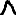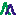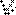Objects Mixture

# Simulation

There is one of these objects for each simulation. The first one is for the main window, with others for each plot window. The settings can all be adjusted from the Constants window, and some can also be adjusted from menus in the main window and text boxes in the main window and plot windows.

## Settings

 IntensityUnits Units for intensity. These are described in detail under Intensity Formulae. Possible values are: Arbitrary - fastest, as it does not include the partition function, but can give misleading results (see below). Normalized - default, and recommended for most cases unless absolute intensities are required. Squared - square the calculated intensity HonlLondon - The line strength factors, essentially the transition dipole moment summed over all the degenerate M levels and squared. The value displayed is actually 3Spol, which is correct for one photon transitions, but see the discussion under Line Strengths for possible caveats for other types of transition. OscillatorStrength - The oscillator strength calculated from the line strength divided by the lower state degeneracy. EinsteinASum - The Einstein A coefficient for each transition summed over upper and lower state M levels, with population factors excluded. This was EinsteinASum up to version 6.0.228. EinsteinA - The Einstein A coefficient for each transition summed over lower state M levels, with population factors excluded. This corresponds to the radiative rate from the upper state. nm2MHzperMolecule - for normal absorption cm2WavenumberperMolecule - for normal absorption HzperMolecule - for emission. This is the rate of emission of photons by one molecule on each transition, including the Boltzmann factor and partition function. See also Emission Spectra for a discussion of fluorescence. WperMolecule - as HzperMolecule, but scaled by hν to give power rather than photons/second. PopDist - Plots an energy diagram, rather than a spectrum, with intensities just the level population. This is intended for modeling a time of flight spectrum or similar experiment where energy levels are observed directly, rather than transitions. NormPopDist - as for PopDist, but include partition function. For the five with units (EinsteinA, nm2MHzperMolecule, nm2MHzperMolecule, HzperMolecule and WperMolecule) and OscillatorStrength, transition moments are assumed to be in Debye for electric dipole transitions and Bohr or nuclear magnetons for magnetic dipole transitions. Misleading results can be obtained for a setting of Arbitrary when simulating isotopologues or isotopomers with different symmetry or statistical weights together, such as 35Cl2 and 35Cl37Cl. The calculation is "correct", in that the partition function is necessary to give the correct relative intensities, but is excluded by design for this setting. LifeModel Model to use for state dependent lifetimes and linewidths. Valid values are lmNone, lmWidth, lmProduct, lmProductWidth, lmParent, lmParentWidth, lmGate, lmGateWidth. See Widths and Lifetime Effects for how this setting works. PlotUnits Units for the horizontal scale for spectrum plots. Possibilities are standard energy units (cm1, MHz, Kelvin and eV) as in the Mixture object and also: sqrtcm1 - Velocity in units of cm-1/2 nmVac - Wavelength in vacuum in nm nmAir - Wavelength in air in nm angVac - Wavelength in vacuum in Å angAir - Wavelength in air in Å The correction between air and vacuum wavelengths is made using the formula for the dispersion of air given in B Edlen, Metrologica, 2, 71 (1966). Fluorescence Set to always discard lower state population; see Emission Spectra for further discussion of this flag. nDF Number of points (between Fmin and Fmax) to calculate the spectrum at. Note that if a peak width is less than 3*(Fmax-Fmin)/nDF, i.e. only a few points wide, then it is shown as a stick, rather than a peak. WidthMult Multiple of line width to extend convolution over. AutoMin If set (the default), Ymin is automatically updated to minimum point in plot range AutoMax If set (the default), Ymax is automatically updated to maximum point in plot range ShowSum Plot overall sum of individual spectra. This is toggled by thebutton. ShowParts Plot individual spectra making up overall spectrum. The individual spectra are grouped by colour, so you will need to set colours to see something different to the sum. Colours can be set at the transition moment, state, molecule or species level. This is toggled by thebutton. ShowFortrat Plot a Fortrat diagram, i.e. J against frequency, in the main window. This is toggled by thebutton. UseUpper Set to use upper rather than lower state J and symmetry in the Fortrat diagram. ShowSymmetry Show symmetry in Fortrat plots. ShowDeltaJ Show change in J in Fortrat plots. ScaleMarkSize Scale Mark Size with intensity in Fortrat plots. UseSymmetry If true, show different symmetries in separate Fortrat plots. UseStateNumber If true, show different state numbers in separate Fortrat plots. FortratQno Select quantum number to use in Fortrat plots. PreserveArea If set, then changing the PlotUnits changes the vertical scale, giving a constant area to peaks. This implies the units of the vertical scale depend on the horizontal scale. For example, if units of cm2WavenumberperMolecule are selected for IntensityUnits and the PlotUnits are MHz the vertical scale units are actually cm2wavenumber/molecule/MHz to give integrated peak areas (calculated from the numerical values plotted) of cm2wavenumber/molecule independent of the PlotUnits. Versions of PGOPHER before 10.1 did this by default. The default in newer versions is to not set this parameter, and thus always plot the same quantity for intensity. Changing the PlotUnits thus does not change the vertical scale, which is less confusing when comparing to experimental spectra or doing contour fits. SeparateScales When showing multiple plots, scale plots separately, rather than requiring the same scale factor for each. LogScale Take ln of vertical axis on plots. (Initial implementation.) Show2DPlot (Future Use) Auto2DY (Future Use)

## Parameters

Several of these can be set from the tool bar in the main window.
 Fmin Left edge of plot range in main window. Fmax Right edge of plot range in main window. Ymin Lower end of current plot range; overwritten with lowest value in current range if AutoMin set Ymax Upper end of current plot range; overwritten with lowest value in current range if AutoMin set Temperature Rotational temperature (Kelvin). Setting this < 0 will force the use of numerical populations for all manifolds, as described in Non-Boltzmann Populations. Gaussian Gaussian contribution to linewidth (full width half maximum). If both this and a Lorentzian width (below) is set the result is a convolution of the two, a Voigt profile. Note that this is in PlotUnits, and is taken to be constant over the plot range, which may be a concern if the plot range is large, as a width that is constant in wavelength will not be constant in  frequency. Lorentzian Lorentzian contribution to linewidth (full width half maximum). This and the Gaussian width can also be set from the main window. Note that this is in PlotUnits, and is taken to be constant over the plot range, which may be a concern if the plot range is large, as a width that is constant in wavelength will not be constant in  frequency. Foffset Frequency offset to simulation. SMargin This setting controls the number of extra points to calculate at each end of the spectrum. (These points are not plotted, but may be required to avoid artefacts in the convoluted spectrum): If > 0: Number of extra points to calculate at each end If < 0: Number of points is |SMargin|*WidthMult*(Gaussian+Lorentzian) OThreshold Ignore peaks smaller than this fraction of the maximum peak intensity in selected simulations. In line listings, lines with an intensity less than this are excluded from the printout; in this case the value is absolute, rather than a fraction of the strongest. Note that line lists produced by File, Export, Line List use a separate value that is a fraction of the maximum intensity. RefWidth Reference width in linewidth (predissociation) calculations; see LifeModel Tvib Vibrational Temperature (Kelvin); set to -1 (default) to use rotational temperature for all Boltzmann factors. MinI Discard lines weaker then this fraction of the strongest in Fortrat plots. Note that the default for this increased from 0 to 0.01 in 10.0.31. Saturation Zero for normal calculation; positive values progressively switch strength to population only by replacing the line strength, S by: S = (1-exp(-S*Saturation/g))*g where g = Min(2J'+1, 2J"+1)*Statistical Weight. This is appropriate for saturation by z polarized light. Values of, say, 1 to 10 will wash out the differences between allowed branches and much higher values will bring out transitions that are only allowed by some weak mixing. Tspin Spin temperature (Kelvin); set to -1 (default) to assume equilibrated nuclear spin states. If set >=0 then the fraction of molecules with each particular nuclear spin species (ortho/para for diatomics) is fixed at the fractions found at T = Tspin. This is often appropriate for molecular beams, where the nuclear spin states do not relax during the expansion. There are two limitations in the current implementation, which may lead to incorrect results: If hyperfine structure is included for equivalent nuclei For asymmetric tops if the statistical weight for two different spin species is the same IScale Scale all simulated intensities by this value. (The default is 1.) EField Static electric field, V/m; see External Fields - The Zeeman and Stark Effects BField Static magnetic field in Tesla (= 104 Gauss); see External Fields - The Zeeman and Stark Effects Doppler Plot each transition as two peaks, split by 2*Doppler*Centre Frequency. This gives the double peak structure often seen in Fourier transform microwave spectroscopy. PlotSplit Fraction of plot area to use for the simulation where simulation and overlay plots are separated. The possible range is -1..1, with 0.5 the default. Negative values put the simulation, rather than the overlay, at the top. Ymin2D (Future Use) Ymax2D (Future Use)

## Operations

Right click on the item in the constants window for the following operations in addition to the standard ones:

 Temperature Table Print a table in the log window of the intensity at the central frequency/wavelength of the simulation. You are prompted for the temperature step size, and the values tabulated will be from 0 to the temperature of the simulation.